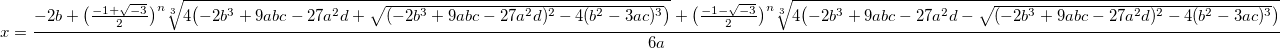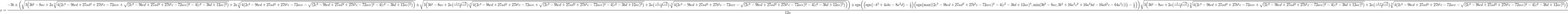# Formulae to Solve of Polynomial Equations

## The Linear FormulaThe linear formula gives the solution of ax + b = 0 for real numbers a, b with a ≠ 0.The quadratic formula gives the solutions of ax2 + bx + c = 0 for real numbers a, b, c with a ≠ 0.

## The Cubic FormulaThe cubic formula gives the solutions of ax3 + bx2 + cx + d = 0 for real numbers a, b, c, d with a ≠ 0.

Directions: Take n = 0, 1, 2. Use real cube roots if possible, and principal roots otherwise.

## The Quartic FormulaThe quartic formula gives the solutions of ax4 + bx3 + cx2 + dx + e = 0 for real numbers a, b, c, d, e with a ≠ 0.

Directions: Choose all possibilities for the three ± signs with the last two equivalent. Use real cube roots if possible, and principal roots otherwise.